# Copper wire

What is the weight of 1000 m copper wire with a diameter of 5 mm when rezistivity p = 8.8 g / cm3?

Result

m =  172.788 kg

#### Solution:Leave us a comment of example and its solution (i.e. if it is still somewhat unclear...):

Showing 0 comments:Be the first to comment!#### To solve this example are needed these knowledge from mathematics:

Do you know the volume and unit volume, and want to convert volume units? Do you want to convert mass units?

## Next similar examples:

1. Aluminum wireAluminum wire of 3 mm diameter has a total weight of 1909 kg and a density of 2700 kg/m3. How long is the wire bundle?
2. Iron cast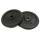What is the weight of cast iron with volume 3575 cubic centimeters. The density of the cast iron is 7600 kg/m3
3. Hydrostatic forceWhat hydrostatic force is applied to an area of 30 cm2 in water at a depth of 20 m? (Water density is 1000 kg/m3)
4. Theorem proveWe want to prove the sentense: If the natural number n is divisible by six, then n is divisible by three. From what assumption we started?
5. Average speed 4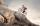Starting at home Tony traveled uphill to the store for 45 minutes at 8 miles per hour. he then traveled back home on the same path at a speed of 24 miles per hour. what is his average speed for the entire trip?
6. ResistanceA resistor having an electrical resistance of 1.5 k ohms passes an electrical current of 0.1 A. Calculate what voltage is between the terminals of the resistor.
7. Plastic pipeCalculate weight of the plastic pipe with diameter d = 70 mm and length 380 cm if the wall thickness is 4 mm and the density of plastic is 1367 kg/m3.
8. Barrel with water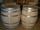The water barrel has a weight of 52 kg. When we pour a quarter of the water, the weight of the barrel is reduced to 40 kg. Determine the weight of the empty barrel.
9. Reservoir + waterReservoir completely filled with water weighs 12 kg. After pouring off three quarters of the amount of water weights 3 kg. Calculate the weight and volume of the reservoir.
10. Potatoes bags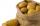I have three bags with 21 kg potatoes. The first bag is 5.5 kg more than in the second bag and the third is 0.5 kg more than in the second bag. Determine how many kgs of potatoes is in each bag.
11. Mass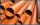The thickness of a metallic tube is 1cm and its outer radius is 11cm. Find the mass of such 1m long tube, if the density of the metal is 7.5g per cubic cm.
12. Water wellDrilled well has a depth 20 meters and 0.1 meters radius. How many liters of water can fit into the well?
13. Cylinder surface areaVolume of a cylinder whose height is equal to the radius of the base is 678.5 dm3. Calculate its surface area.
14. 6 termsFind the first six terms of the sequence. a1 = 7, an = an-1 + 6
15. Nineteenth memberFind the nineteenth member of the arithmetic sequence: a1=33 d=5 find a19
16. Volleyball8 girls wants to play volleyball against boys. On the field at one time can be six players per team. How many initial teams of this girls may trainer to choose?
17. Collecting day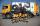At collecting day at school pupils of three classes took total of 1063 kg of paper. Class B collected half more than A Class and class C 55 kg more than B. How many kg of paper collected each class?